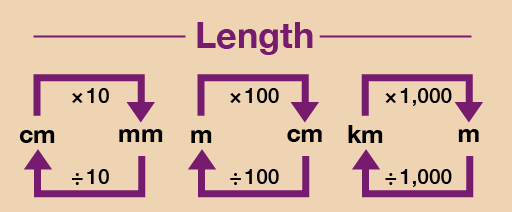Everyday maths 1 (Wales)

Start this free course now. Just create an account and sign in. Enrol and complete the course for a free statement of participation or digital badge if available.

Free course

# 1.4 Converting units

You may often need to convert between different units of length. For example, if you were fitting a kitchen or measuring a piece of furniture, you might need to convert between millimetres and centimetres, or centimetres and metres.

Figure 9 shows you how to convert between metric units of length.Figure 9 A conversion chart for length

Hint: To convert from a bigger unit to a smaller unit (such as cm to mm), you multiply. To convert from a smaller unit to a bigger unit (such as mm to cm), you divide.

## Example: Converting units of length

1. What is 8.5 metres in centimetres?
2. What is 475 centimetres in metres?

### Method

1. Converting between metric units involves multiplying or dividing by 10, 100 or 1 000, which you will have practised in Session 1. As you can see from Figure 9, you need to multiply by 100 to convert from metres (m) to centimetres (cm). So converting 8.5 m into centimetres would be:
• 8.5 m × 100 = 850 cm
2. As you can see from Figure 9, you need to divide by 100 to convert from centimetres (cm) to metres (m). So converting 475 cm into metres would be:
• 475 cm ÷ 100 = 4.75 m

Now try the following activities.

## Activity 5: Converting lengths

Please work these out without using a calculator. You may wish to look back at Session 1 first to remind you how to multiply [Tip: hold Ctrl and click a link to open it in a new tab. (Hide tip)] and divide by 10, 100 and 1 000.

1. 20 mm = ? cm
2. 54 mm = ? cm
3. 0.5 cm = ? mm
4. 8.6 cm = ? mm
5. 400 cm = ? m
6. 325cm = ? m
7. 12 m = ? cm
8. 6.8 m = ? cm
9. 450 mm = ? m (Hint: You will need to look at the chart for how to convert from millimetres to centimetres and then centimetres to metres)
10. 2 m = ? mm
11. 8 km = ? m
12. 500 m = ? km
13. I am 1.6 m tall. How tall am I in centimetres?
14. You are fitting kitchen cabinets. The gap for the last cabinet is 80 cm. The sizes of the cabinets are shown in millimetres. Which size should you look for?
15. You want to buy 30 cm of fabric. The fabric is sold by the metre. What should you ask for?

1. 20 mm ÷ 10 = 2 cm
2. 54 mm ÷ 10 = 5.4 cm
3. 0.5 cm × 10 = 5 mm
4. 8.6 cm × 10 = 86 mm
5. 400 cm ÷ 100 = 4 m
6. 325 cm ÷ 100 = 3.25 m
7. 12 m × 100 = 1 200 cm
8. 6.8 m × 100 = 680 cm
9. There are 10 mm in 1 cm, so divide by 10 first to convert 450 mm to 45 cm. There are 100 cm in 1 m, so divide 45 cm by 100 to get the answer, 0.45 m.
10. There are 100 cm in 1 m, so multiply by 100 first to convert 2 m to 200 cm. There are 10 mm in 1 cm, so multiply 200 cm by 10 to get the answer, 2 000 mm.
11. 8 km × 1 000 = 8 000 m
12. 500m ÷ 1 000 = 0.5 km
13. There are 100 cm in 1 m, so to convert from metres to centimetres you need to multiply by 100:
• 1.6 m × 100 = 160 cm
14. To convert from centimetres to millimetres, you need to multiply the figure in centimetres by 10. The size is 80 cm, so the answer is:
• 80 × 10 = 800 mm
15. To convert from centimetres to metres, you need to divide the figure in centimetres by 100. The length of fabric you need is 30 cm, so the answer is:
• 30 ÷ 100 = 0.3 m

## Activity 6: Matching the same measurement

Match the following measurements:

Using the following two lists, match each numbered item with the correct letter.

1. 5 m

2. 15 mm

3. 150 mm

4. 50 mm

5. 0.5 km

6. 150 cm

7. 15 m

8. 1.5 km

• a.1 500 m

• b.15 cm

• c.5 cm

• d.1 500 cm

• e.1.5 cm

• f.1.5 m

• g.500 m

• h.500 cm

• 1 = h
• 2 = e
• 3 = b
• 4 = c
• 5 = g
• 6 = f
• 7 = d
• 8 = a
FSM_1_CYMRU#### Live in Wales? Free your ambition with a paid part-time course with the OU in Wales.

With grants of up to £4,500* to help with living costs, and tuition fee loans to cover course fees,
now's the time to take that next step.

#### Find out more

*Eligibility rules apply for financial support.